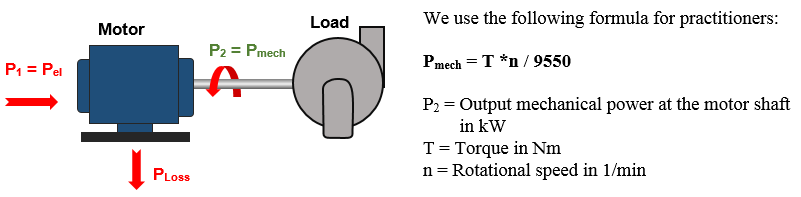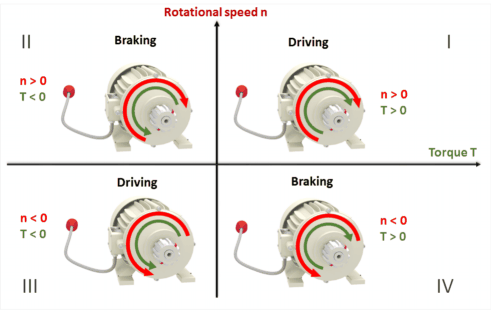## Electrical motors as energy converter

Electric motors convert electrical energy into mechanical energy. The engine drives a work machine and thus converts electrical energy into mechanical energy. Depending on the voltage, DC and AC machines are used in practice.

Criterias for the selection of an electric motor

• Power requirement of the working machine
• operating time and switching frequency
• Current type, mains voltage and mains frequency
• Speed and direction of rotation
• Size typemotor as an energy converter

The power loss has several reasons:

• Friction losses in the bearings and eventually the brushes
• Iron losses due to eddy currents and hysteresis losses
• Loss of excitation due to changes in the magnetic field
• Loss of the motor winding (ohmic and inductive resistance)

The efficiency η of an electric motor is given by the ratio of the mechanical output power Pmech (P2) to the electric input power Pelectr. (P1).

ƞ = P2 / P1  and PV = P1 - P2